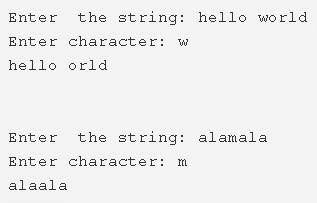# C Program To Remove First Occurrence Of A Character From String

C program to remove the first occurrence of a character from a string – In this article, we will brief in on the numerous methods to remove the first occurrence of a character from a string in C programming.

Suitable examples and sample programs have also been added so that you can understand the whole thing very clearly. The compiler has also been added with which you can execute it yourself.

The methods used in this piece are as follows:

• Using Standard Method
• Using Function
• Using Recursion

A string is nothing but an array of characters. The value of a string is determined by the terminating character. Its value is considered to be 0.As you can see in the example given above, firstly, you need to enter the specified string of your choice.

The string entered here is as follows:

“hello world”

Then, you need to particularise a character which you want to remove on its first occurrence.

The character ‘w’ is chosen for this string. Hence, the new string looks like this after ‘w’ is removed:

“hello orld”

Thus, the ways to do the same in C programming are as follows:

## Using Standard Method

1. Read the entered string and store the string into the character array variable ‘s’ using gets(s).

2) Read the entered character using getchar() and store the character in the variable c.

3) Iterate the first for loop till the end of the character of the string with the structure for(i=0;s[i];i++).

Then initialize the i value to n. Here i indicates the length of the string.

5) If the character is equal to the element of the string, then shift the elements of the string which are right to the removed element, to one position left.

6) Print the string after removing the first occurrence of the character from the string.

Output:

### Using Function

1. The main() function calls the stringlength(char *s) by passing the string as an argument.

2) The stringlength(char *s) returns the length of the string.

3) Then function deletechar(char *s, char c) will remove the first occurrence of the character from the string.

temp=1.

For loop iterates through the string until the end of the string.

a) if temp=1

If the entered character is equal to the element of the string then

Initialize the temp=0 and shift the element right to the removed element to one position left.

b) If temp!=1 then shift the next element to the shifted element to one position left.

After all the iterations of the for loop, we will get the string with the characters except the character which we want to remove.

Output:

#### Using Recursion

1. The function stringlength(char *s) returns the length of the given string.

2) temp=1,i=0.

If i< the length of the string

a) if temp=1

If the entered character is equal to the element of the string then temp=0 and shift the element right to the removed character to one position left.

b) if temp!=1 then shift the next element to the shifted element to one position left.

Increase the i value by 1.

Call the function itself by taking the modified string, character to be removed as arguments. The function calls itself recursively until i<length of the string becomes false.

Output:

x

## C Program To Print Number Of Days In A Month | Java Tutoring

C program to input the month number and print the number of days in that ...